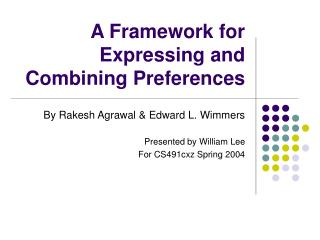DownloadDownload PresentationA Framework for Expressing and Combining Preferences

# A Framework for Expressing and Combining Preferences

Télécharger la présentation## A Framework for Expressing and Combining Preferences

- - - - - - - - - - - - - - - - - - - - - - - - - - - E N D - - - - - - - - - - - - - - - - - - - - - - - - - - -
##### Presentation Transcript

1. A Framework for Expressing and Combining Preferences By Rakesh Agrawal & Edward L. Wimmers Presented by William Lee For CS491cxz Spring 2004

2. Problem • Explosion of information (the web) • Impossible to construct a “social preference function” without leaving out individual preferences

3. Related Work • Recommendation systems • E.g. Personalogic (no longer in business?) • Problem • Solutions are too specific -- needs a more generic preference framework

4. combining preferences The Preference Problem Model # Any color Alice’s preference function A0 Model # Best Choice: Model: 1 Color: Black Quality: Good Quality Betty’s preference function B0

5. Record Type • Record type is set of (name : type) pair that is used to express the preference parameters. • Examples: • dom(A0)={model: int, color: string union {*}} • dom(B0)={model: int, quality: int}

6. ScoreBoard • ScoreBoard(rt) is by itself a record type! • Define the data type to store Scores • New names of the record type = all combinations of the wild types in the original rt separated with “!”. • 2^j number of fields, j = number of wild types • Use “star!” for the wild type • Examples • ScoreBoard(dom(A0))={color!model: score, star!!model: score} • ScoreBoard(dom(B0))={model!quality: score}

7. RecordOfrt(r,n’1! n’2!...n’k) • RecordOf() a particular record always results in a generalization of the record. • Example • If r = {color = “red”, model = 134} • RecordOfdom(A)(r,star!!model)={color = *,model = 134} (a more general r based on the field of the ScoreBoard)

8. Scores • Scores(p,r) applies the preference function p to the generalization of record r • Scores(p,r).n’1!...n’k=p(RecordOfrt(r, n’1!...n’k)) • Example: • Scores(A0,r)={color!model = 0.3, star!!model = 0.5} • Where A0({color = r.color, model=r.model})=0.3 and A0({color=*, model=r.model})=0.5

9. Putting Everything Together r = record: {color: white model: 1342 quality: 3} Field name: star!!model rt = record type: {color: white or * model: int} RecordOfrt(r,star!!model): color: * model: 1342 ScoreBoard(rt): {color!model: score, star!!model: score Scores(A0,r): {color!model: veto, star!!model: 0.3} Scores(B0,r): {color!quality: 0.5, star!!quality: 0.4} A0 (preference function that has type rt) (combine(f))(a: Scores(A0,r), b: Scores(B0,r), r) = final score

10. Example Value Function (f) • Alice has the veto power to veto a color/model combination or on a particular model. If she thinks that it’s OK, then let Betty’s “quality” decide. FirstVeto(a: ScoreBoard(dom(A0)), b: ScoreBoard(dom(B0)), c: merge(dom(A0), dom(B0))) returns score { if a.color!model = veto then return veto else if a.star!!model = veto then return veto else return b.model!quality }

11. Implementation using RDBMS Alice’s preference function • Preference functions (p, or A0 or B0) are implemented in a table Betty’s preference function

12. Generating the Scores • To generate the “Scores” for Alice: • color!model: = • SELECT Score FROM Alice WHERE Color = r.color AND Model = r.model • Return score, or NO_PREF if query returns nothing • star!!model = • SELECT Score FROM Alice WHERE Color = * AND Model = r.model • Similarly for Betty, model!quality = SELECT Score FROM Betty WHERE Model = r.model AND Quality = r.quality

13. Combining the Scores • Get the Scores for Alice • Get the Scores for Betty • Returns the value obtained by FirstVeto(a,b,r) • The FirstVeto function can be realized by a table with 4 columns (Color, Model, Quality, and Score) that covers the domain of the records that we pass to it.

14. Questions for Discussion • What about the running time? • 1000 fridges = 1000 * (2^j) SELECTs for each user where j is the number of fields that have type *. • Would this be a problem? • Does this system works on web search? (Think Alice == Google and Betty == you) • How can the system learn the user’s preference function?

15. Thanks!